HOTS Questions: Motion

# HOTS Questions: Motion - Class 9

Test Description

## 25 Questions MCQ Test Science Class 9 - HOTS Questions: Motion

HOTS Questions: Motion for Class 9 2023 is part of Science Class 9 preparation. The HOTS Questions: Motion questions and answers have been prepared according to the Class 9 exam syllabus.The HOTS Questions: Motion MCQs are made for Class 9 2023 Exam. Find important definitions, questions, notes, meanings, examples, exercises, MCQs and online tests for HOTS Questions: Motion below.
Solutions of HOTS Questions: Motion questions in English are available as part of our Science Class 9 for Class 9 & HOTS Questions: Motion solutions in Hindi for Science Class 9 course. Download more important topics, notes, lectures and mock test series for Class 9 Exam by signing up for free. Attempt HOTS Questions: Motion | 25 questions in 25 minutes | Mock test for Class 9 preparation | Free important questions MCQ to study Science Class 9 for Class 9 Exam | Download free PDF with solutions
 1 Crore+ students have signed up on EduRev. Have you?
HOTS Questions: Motion - Question 1

### A body moves in a uniform circular motion

Detailed Solution for HOTS Questions: Motion - Question 1
Accelerating objects are objects which are changing their velocity - either the speed (i.e., magnitude of the velocity vector) or the direction. An object undergoing uniform circular motion is moving with a constant speed. Nonetheless, it is accelerating due to its change in direction.
HOTS Questions: Motion - Question 2

### 180° plane angle is equal to

Detailed Solution for HOTS Questions: Motion - Question 2

π radian is equal to 180 ° . Radian is a unit of measurement for angles, just like degrees are, and π is just the no. of radians that makes up that angle.

HOTS Questions: Motion - Question 3

### Speed of a body in particular direction can be called

Detailed Solution for HOTS Questions: Motion - Question 3
Distance covered by a body in per unit time is called Speed & Distance covered by a body in a given direction is known as Velocity ....... It can also be written as speed of a body in a particular direction is called Velocity .
HOTS Questions: Motion - Question 4

Statement A: Uniform circular motion is a case of accelerated motion
Statement B: In third equation of motion we do not have the time.

Detailed Solution for HOTS Questions: Motion - Question 4

Uniform circular motion is always accelerated because the direction of velocity is continuously changing hence velocity is changing which causes change in acceleration. Hence the given statement is true.
There are 3 equations of motion,

• v = u+ at
• s = ut + 1 /2 at
• v2 = u2 + 2as

As, we can see there , we don't have the time in the third equation of motion.

HOTS Questions: Motion - Question 5

Which of the following is correct about uniform circular motion?
A. Direction of motion is continuously changed
B. Direction of motion is not changed
C. Speed and direction both remain constant
D. Speed is constant, but direction is changing

Detailed Solution for HOTS Questions: Motion - Question 5

Uniform circular motion is accelerated because the velocity changes due to continuous change in the direction of motion. So, even when the body moves ta a constant speed, its velocity is not constant. Thus, for a body moving in a circular path at a constant speed, its velocity changes continuously. The change in velocity gives rise to an acceleration in the moving body. Therefore circular motion is an acceleration motion even when the speed remains constant.

HOTS Questions: Motion - Question 6

Which of the quantities – speed, time, velocity and acceleration have the same SI unit?

Detailed Solution for HOTS Questions: Motion - Question 6

The SI unit is the International System of Units, which is a system of measurement used in science and engineering. In this question, we need to determine which quantities have the same SI unit.
To answer this question, let's analyze each quantity one by one:
Speed: Speed is the rate at which an object covers a distance. It is defined as the distance traveled divided by the time taken. The SI unit of speed is meters per second (m/s).
Time: Time is a scalar quantity that measures the duration between two events. The SI unit of time is seconds (s).
Velocity: Velocity is a vector quantity that measures the rate at which an object changes its position with respect to time. It includes both magnitude (speed) and direction. The SI unit of velocity is also meters per second (m/s).
Acceleration: Acceleration is the rate at which an object changes its velocity. It is defined as the change in velocity divided by the time taken. The SI unit of acceleration is meters per second squared (m/s^2).
Now, let's compare the SI units of each quantity:
- Speed and velocity both have the same SI unit, which is meters per second (m/s). Therefore, option A is correct.
- Time has the SI unit of seconds (s), which is different from the SI units of speed, velocity, and acceleration.
- Acceleration has the SI unit of meters per second squared (m/s^2), which is different from the SI units of speed, velocity, and time.
Therefore, the correct answer is option A: speed and velocity have the same SI unit.
HOTS Questions: Motion - Question 7

Find the correct statement

Detailed Solution for HOTS Questions: Motion - Question 7

When the velocity decreases with respect to time it is termed as retardation or deceleration. In reality negative acceleration is commonly called retardation. Its SI unit. is m/s2.

HOTS Questions: Motion - Question 8

A body is said to be in rest when

Detailed Solution for HOTS Questions: Motion - Question 8
If philosophically speaking one could be physically at rest but mentally in motion for example when one is taking a nap, except one is dead, one's internal system is constantly in motion as breathing occurs, dreams, digestion etc. Rest is a state in physics that refers to an object being stationary relative to a particular frame of reference or another object; when the position of a body with respect to its surroundings does not change with time it is said to be "at rest".
HOTS Questions: Motion - Question 9

A bus moves from stop ‘A to stop ‘B’ with a speed of 40 km/hr and then from stop ‘B’ to stop ‘A with a speed of 50 km/hr. Its average speed is

Detailed Solution for HOTS Questions: Motion - Question 9

Let the distance between A and B be D and time taken to cover the distance be T1 and T2.

Speed = Distance/Time

40 = (D/T1) and 50 = (D/T2)

T1 = (D/40)

also, T2 = (D/50)

Total time (T) = T1 + T2

⇒ (D/40) + (D/50) = (9D/200)

Total Distance = (D + D) = 2D

Average speed = Total Distance/Total Time Taken

= (2D/(9D/200)) = 400/9 = 44.4 km/hr

HOTS Questions: Motion - Question 10

Find the incorrect statement

Detailed Solution for HOTS Questions: Motion - Question 10

When a body moves with constant speed its acceleration is zero . The body is said to be accelerating if it moves in a uniform circular motion. The slope of the velocity- time graph gives Instantaneous acceleration . All the given statements are correct. Therefore ,  option ( d) is correct answer.

HOTS Questions: Motion - Question 11

Match the following with correct response.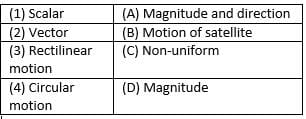HOTS Questions: Motion - Question 12

Match the following with correct response.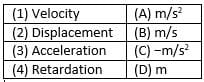HOTS Questions: Motion - Question 13

Match the following with correct response.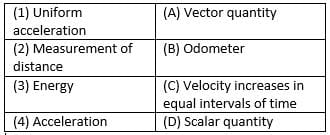Detailed Solution for HOTS Questions: Motion - Question 13

Vector- A physical quantity having a magnitude as well as direction is known as vector quantity.
Odometer - an instrument for measuring the distance travelled by a wheeled vehicle.
Acceleration of a body is defined as the rate of change of its velocity with time.
Scalar - A physical quantity having only magnitude is known as a scalar quantity.

HOTS Questions: Motion - Question 14

The area under the velocity – time graph gives the

Detailed Solution for HOTS Questions: Motion - Question 14

The correct answer is B as velocity-time graph we will get displacement travelled.as ,displacement=velocity/time

HOTS Questions: Motion - Question 15

Which of the following is a false statement

Detailed Solution for HOTS Questions: Motion - Question 15
A body can have a constant speed but a changing velocity because the direction can change while the speed is constant. (A car goes 50 mph around a roundabout). However, a body can not have a constant velocity with a changing speed.
HOTS Questions: Motion - Question 16

Is the following graph possible ?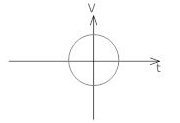Detailed Solution for HOTS Questions: Motion - Question 16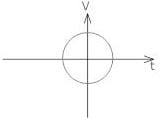The graph shown above is not possible in case of Velocity - time graph. Graph may be straight  curved but not completely circular.

HOTS Questions: Motion - Question 17

Which of the following situations are not possible?

Detailed Solution for HOTS Questions: Motion - Question 17

Yes, a body can have a constant speed and still be accelerating.
Acceleration can be either due to change in speed or due to direction of motion or both.
Consider an example of a uniform circular motion. In a uniform circular motion, the body moves with a constant speed but we still say that it is accelerating due to the change in direction
This acceleration is called centripetal acceleration and is given as
a​= −v2/r​
where v= constant speed

HOTS Questions: Motion - Question 18

If we get a curve line in displacement – time graph then the velocity is

Detailed Solution for HOTS Questions: Motion - Question 18
Explanation:

If we get a curve line in the displacement – time graph, it means that the velocity of the object is changing over time. The velocity is not constant but varies at different points on the curve.

Reasoning:

A curve in the displacement – time graph indicates that the object is undergoing acceleration, which in turn means that the velocity is changing. The shape of the curve can provide information about the nature of the change in velocity.

Different Cases:

There are different cases when we can have a curve in the displacement – time graph:

• Increasing velocity: If the curve is upward sloping, it means that the velocity is increasing with time.

• Decreasing velocity: If the curve is downward sloping, it means that the velocity is decreasing with time.

• Changing acceleration: If the curve has varying slopes, it means that the acceleration is changing, and therefore, the velocity is also changing.

Conclusion:

Therefore, if we get a curve line in the displacement – time graph, the velocity is non-uniform, as it is changing over time. Option B is the correct answer.

HOTS Questions: Motion - Question 19

Match the following with correct response.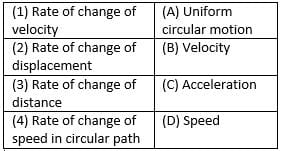HOTS Questions: Motion - Question 20

Match the following with correct response.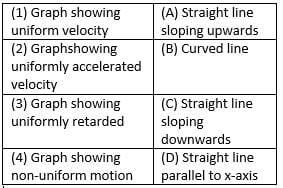HOTS Questions: Motion - Question 21

Which of the following statement is correct?
A. When a body starts from rest, u = 0
B. When a body comes to rest, V = 0
C. For a body under free fall, a = −9.8m/s2
D. For uniform velocity, a = 0

Detailed Solution for HOTS Questions: Motion - Question 21

Acceleration is positive in the “down” direction, where “down” is where the nearest big source of gravity is.

In physics, acceleration is usually expressed as a vector, i.e. a direction and a magnitude. A magnitude is an absolute value, hence always positive.

HOTS Questions: Motion - Question 22

The slope of distance – time graph gives instantaneous.

Detailed Solution for HOTS Questions: Motion - Question 22
The gradient of a distance-time graph represents the speed of an object. The velocity of an object is its speed in a particular direction. The slope on a velocity-time graph represents the acceleration of an object. The distance travelled is equal to the area under a velocity-time graph.
HOTS Questions: Motion - Question 23

Distance is a __________ quantity

Detailed Solution for HOTS Questions: Motion - Question 23

Distance is a scalar quantity that refers to "how much ground an object has covered" during its motion.

HOTS Questions: Motion - Question 24

If a body doesn't rotates then its motion is

Detailed Solution for HOTS Questions: Motion - Question 24
The correct answer is B because this applies in the case of inertia. if you are travelling on a vehicle you are not doing and movement but the vehicle is moving then at that time you are at rest as well as at motion.
HOTS Questions: Motion - Question 25

A body is moving with a velocity of 10m/s. If the motion is uniform, what will be the velocity after 10 second?

Detailed Solution for HOTS Questions: Motion - Question 25

As the body is moving in uniform motion, so the acceleration will be zero.
as acceleration is zero, the change in velocity is also zero.it means velocity will be the same in whole motion.

## Science Class 9

66 videos|352 docs|97 tests
Information about HOTS Questions: Motion Page
In this test you can find the Exam questions for HOTS Questions: Motion solved & explained in the simplest way possible. Besides giving Questions and answers for HOTS Questions: Motion, EduRev gives you an ample number of Online tests for practice

## Science Class 9

66 videos|352 docs|97 tests